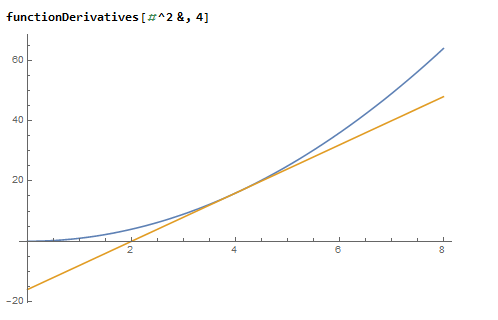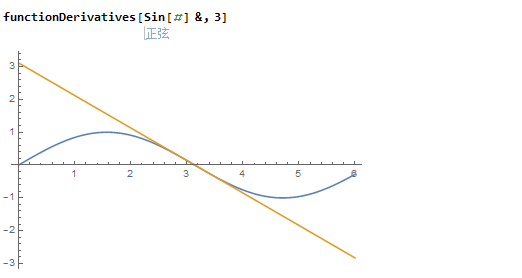# Mathematica画任意函数某点处切线

• A+

## 代码

``functionDerivatives[f_, x_] := Plot[{f[t], f'[x] (t - x) + f[x]}, {t, 0, 2 x}]``• 微信公众号
• 关注微信公众号
•• QQ群
• 我们的QQ群号
•